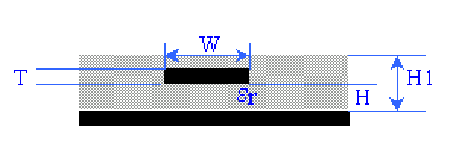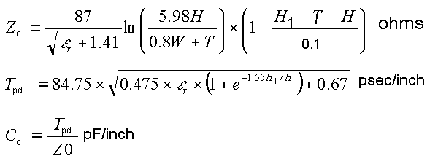### Embedded Microstrip Impedance CalculatorProvide values for the four parameters H1, H, T, W, and the relative permittivity of the dielectric. Click the button corresponding to the characteristic impedance to calculate its value. ( 0.1< W/H < 3.0; 1 < εr < 15 )

Select the units for all dimensions: mm mils
 H1 (Dielectric thickness) : mm mils H (Height above reference plane): mm mils T (Trace thickness): mm mils W (Trace width): mm mils εr (Relative permittivity): (Characteristic impedance): ohms C0 (Capacitance): pF/cm pF/inch Tpd (Propagation delay time): psec/cm psec/inch

Notes:

• The formulas used here are taken from the Design Guide for Electronic Packaging Utilizing High-Speed Techniques (4th Working Draft, IPC-2251, February 2001)• Range of valid parameters specified in the Design Guide: 0.1< W/H < 3.0; 1 < εr < 15;
• Note the equations used here are proposal currently being evaluated by IPC. The embedded microstrip impedance formula is an approximation and the results are highly sensitive to the values of the variables. The accuracy of the equations was observed to be slightly better for higher permittivities.
• For typical PCB parameters (εr = 4, H1 = 61.37 mil, H = 30 mil and T = 1.37 mil), the deviation of the calculated results obtained using XFX, a 2D numerical field solver from Innoveda, is listed below:

 Zo: 0.1 < W/H < 1.0 within 20% 1.0 < W/H < 3.0 20%--40% Co: 0.1 < W/H < 1.0 within 5% 1.0 < W/H < 2.2 within 20% 2.2 < W/H < 3.0 20%--40%